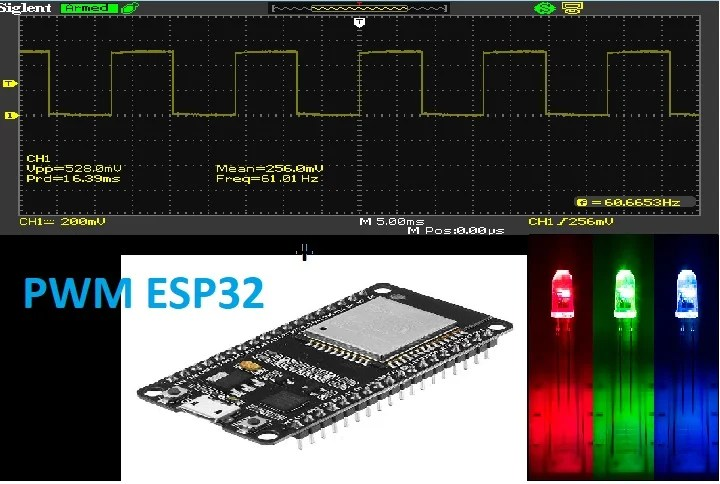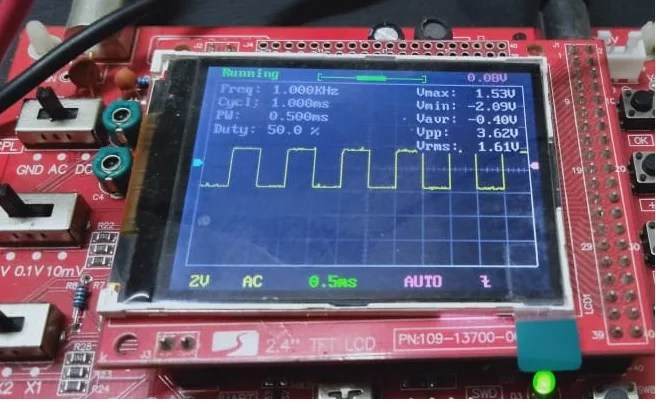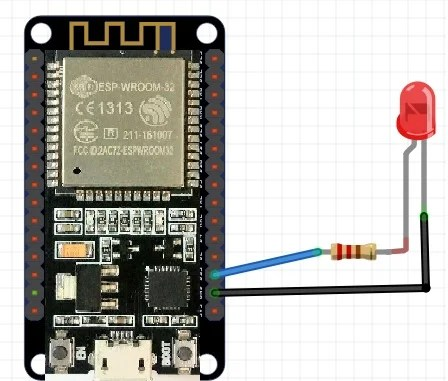# ESP32 PWM with Arduino IDE – LED fading example

In this ninth tutorial on ESP32 development board, We will talk about PWM or pulse width modulation pins of ESP32 development board using ESP32 DOIT devkit. You will see how to produce PWM using GPIO pins of this development board.  All GPIO pins of this development board can be used to get the PWM signal. We only need to specify a PIN on which we want to get a PWM signal.Like other built-in modules ADC, Touch panels, hall effect sensors, ESP32 board also has integrated PWM controller in it.  It supports sixteen pulse width modulation channels. We can use these channels to get PWM signal from any GPIO pin. Before that, we need to configure its parameters and PIN assignment. We will that shortly how to set these parameters. In this tutorial, you will learn to use about PWM for different applications:

• First you will learn how to configure these channels to generate signals on ESP32 pins.
• You will learn how to generate fix duty cycle signal.
• You will also see how to generate variable duty cycle signal.
• At the end, I will show you an example of LED fading with GPIO pin 15 as a digital control signal.

Followings are the mandatory backgrounds concepts to completely understand this tutorial:

## How to configure PWM channels of ESP32

If you know Arduino Uno programming, you must see that the analogWrite function is used to generate PWM in Arduino IDE for Arduino related board. But a different function is used to produce PWM signal for ESP32 in Arduino IDE.  Follow these step to set parameters for all channels of pulse width modulation:

• There are 16 PWM channels available. You need to choose any channel between 0 and 15.
• The second step is to choose the frequency of the digital signal.  For example, you can set the frequency like 10,000 hertz, 5000 hertz or any other value you want.
• ESP32 boards support PWM resolution between 1 bit to 16 bits. But remember the frequency and resolution of the PWM signal has an inverse effect on each other. So to achieve maximum frequency, the optimal resolution is 8 bit.  You can find more discussion on it in this link.
• We will be using 8-bit resolution, and duty cycle value will vary between 0-255. Duty cycle defines the width of the signal or on time of the signal.
• The last step is to attach the GPIO PIN with a PWM channel of your own choice.

Now let’s see how to set these parameters inside the PWM library. Three functions are used for this purpose. One is used to set the PIN with the channel. The second function is used to set the frequency,  resolution, and channel of the PWM controller and the third function is used to generate the signal with a specified duty cycle.

• ledcSetup(PWM_Channel_Number, Frequency, resolution): You should pass three arguments as an input to this function,  channel number, frequency and the resolution of PWM channel. We use this function inside the setup function only.
• ledcAttachPin(GPIO_PIN , CHANNEL) : This function accepts two arguments. One is the GPIO pin on which we want to get the OUTPUT of signal and second argument is the channel which we produce the signal.
• ledcWrite(CHANNEL, DUTY_CYCLE): ledcWrite function is used to generate the signal with a duty cycle value.

## Pulse width modulation example with ESP32Now we will see an example to generate a pulse width modulation signal on any GPIO pin, and I will show you signal on the oscilloscope. But the same case can be used as a dimming LED example, or fading LED example.  First, we will see an example with fix duty cycle signal on the oscilloscope, and after that, we will see an example of variable duty cycle PWM which can also be used as led dimming example.

I will be using GPIO15 to get this signal and will show this signal on the oscilloscope.  Now you just need to connect oscilloscope positive terminal with GPIO pin fifteen and ground terminal of the oscilloscope with the ground of the board.  Now, this simple code will generate a PWM signal with a duty cycle of 50%.

``````int PWM_FREQUENCY = 1000; // this variable is used to define the time period
int PWM_CHANNEL = 0; // this variable is used to select the channel number
int PWM_RESOUTION = 8; // this will define the resolution of the signal which is 8 in this case
int GPIOPIN = 15 ; // GPIO to which we want to attach this channel signal
int dutyCycle = 127; // it will define the width of signal or also the one time

void setup()
{

ledcSetup(PWM_CHANNEL, PWM_FREQUENCY, PWM_RESOUTION);
ledcAttachPin(GPIOPIN, PWM_CHANNEL);

}

void loop()
{

ledcWrite(PWM_CHANNEL, dutyCycle);

}``````

This is a simple code to generate a pulse width modulation signal with the duty cycle of 50%. In these line of program, we have define the parameters of the wave form. First we define the frequency of 1000 hertz. We have selected channel zero. But you can select any channel you want from a total of sixteen channels. After that, we specified the resolution of 8 bit. But you can choose any resolution you want.  We will be using general purpose input output pin 15 to get the output. At the end of the initialization section, we set the duty cycle to 127. In this example, we are getting a waveform with fix duty cycle.

``````int PWM_FREQUENCY = 1000;
int PWM_CHANNEL = 0;
int PWM_RESOUTION = 8;
int GPIOPIN = 15 ;
int dutyCycle = 127;``````

Now in the setup function, we need to use two function ledcSetup and ledcAttach which I have explained above.  First procedure will select the channel zero along with the frequency of 1000 hertz and resolution of 8 bits. With 8 bit resolution, we can specify the duty cycle between 0 and (2^8-) = 255. So if we use 255 as a duty cycle parameter, the duty cycle will be 100% and with 127 duty cycle will be 50% and similarly for other values. The second statement will attach the pulse width modulation channel zero to digital pin fifteen.

``````ledcSetup(PWM_CHANNEL, PWM_FREQUENCY, PWM_RESOUTION);
ledcAttachPin(GPIOPIN, PWM_CHANNEL);``````

Inside the loop function, we are using only instruction which is to generate a signal with a duty cycle of 127 and you will see it will generate a duty cycle of 50%. The waveform will be high for half of the time and logical low for half of the time and total time period will be inverse of the signal’s frequency.  So this ledcWrite function is used to produce PWM signal with a specific duty cycle. you only need to define channel number and duty cycle value as an argument to this function.

``ledcWrite(PWM_CHANNEL, dutyCycle);``

So When you upload this code to your board and connect oscilloscope across the PIN 15, which types of signal you will get as an output? You will see the digital waveform with the following parameters:

• Frequency = 1000 Hertz
• Time period = 1 ms
• duty cycle = 50%
• on time = 0.5 ms
• off time = 0.5 ms

As you can see in the picture below.You have now learned how to generate PWM with a required duty cycle. Now we will see the example of creating a variable PWM signal with ESP32 and how we use it for led fading example.

## Variable duty cycle PWM with ESP32

If your concept is not clear about pulse width modulation, you must be wondering what is meant by the variable duty cycle signal? Variable duty cycle means we want to have digital waveform at the output whose duty cycle will increase from low to high value with some delay. For instance, when we turn on the development board, initially duty cycle will be zero and then it will start increasing with a step of one with some delay. It is very easy to accomplish this task. you just have to change the value of duty cycle inside the ledcWrite function. So the rest of the code which we used in the last example will remain the same except the variable duty cycle part instead of fix. Code for this example is given here.

``````int PWM_FREQUENCY = 1000;
int PWM_CHANNEL = 0;
int PWM_RESOUTION = 8;
int GPIOPIN = 15 ;

void setup()
{

ledcSetup(PWM_CHANNEL, PWM_FREQUENCY, PWM_RESOUTION);
ledcAttachPin(GPIOPIN, PWM_CHANNEL);

}

void loop()
{

for (int dutyCycle = 0; dutyCycle <= 255; dutyCycle++)
{
ledcWrite(PWM_CHANNEL, dutyCycle);
delay(100);
}
}``````

the code is similar to the last program except for the loop function part. Inside the loop part, another for loop is used. Inside for loop, starting value of duty cycle is zero and its value keeps increasing with an increment of one with each iteration of for loop. Each iteration occurs after a delay of 100 milliseconds. So you will observe the signal on an oscilloscope with increasing order of pulse width.  you can see results in these picture of different pulse widths.For LED fading example, you can simply connect an LED with pin number 15 of ESP32 development board and you can upload the same code of variable pulse width to the board. you will see the brightness of LED will be very low at the start and then it start increasing. After some time, LED will glow fully.

you might be interested in other ESP32 tutorials and projects:

### 3 thoughts on “ESP32 PWM with Arduino IDE – LED fading example”

1. Is there a library (.h) file attached to this process? I put the code in so I could do a PWM LED output and Arduino kicks it back because it doesn’t recognize the code. I dont get color changes in the code indicating it sees it differently.

2. Hello
I would like to help me. Thanks a lot in advance.
After I tried to verify your code I have got the following error:

Arduino: 1.8.9 (Windows 7), Board: “NodeMCU 1.0 (ESP-12E Module), 80 MHz, Flash, Disabled, All SSL ciphers (most compatible), 4M (no SPIFFS), v2 Lower Memory, Disabled, None, Only Sketch, 115200”

C:\Users\Prompt\Documents\Arduino\Test_PWM_ESP32\Test_PWM_ESP32.ino: In function ‘void setup()’:

Test_PWM_ESP32:10:52: error: ‘ledcSetup’ was not declared in this scope

ledcSetup(PWM_CHANNEL, PWM_FREQUENCY, PWM_RESOUTION);

^

Test_PWM_ESP32:11:35: error: ‘ledcAttachPin’ was not declared in this scope

ledcAttachPin(GPIOPIN, PWM_CHANNEL);

^

C:\Users\Prompt\Documents\Arduino\Test_PWM_ESP32\Test_PWM_ESP32.ino: In function ‘void loop()’:

Test_PWM_ESP32:19:33: error: ‘ledcWrite’ was not declared in this scope

ledcWrite(PWM_CHANNEL, dutyCycle);

^

exit status 1
‘ledcSetup’ was not declared in this scope